# 半监督学习

07/02 08:57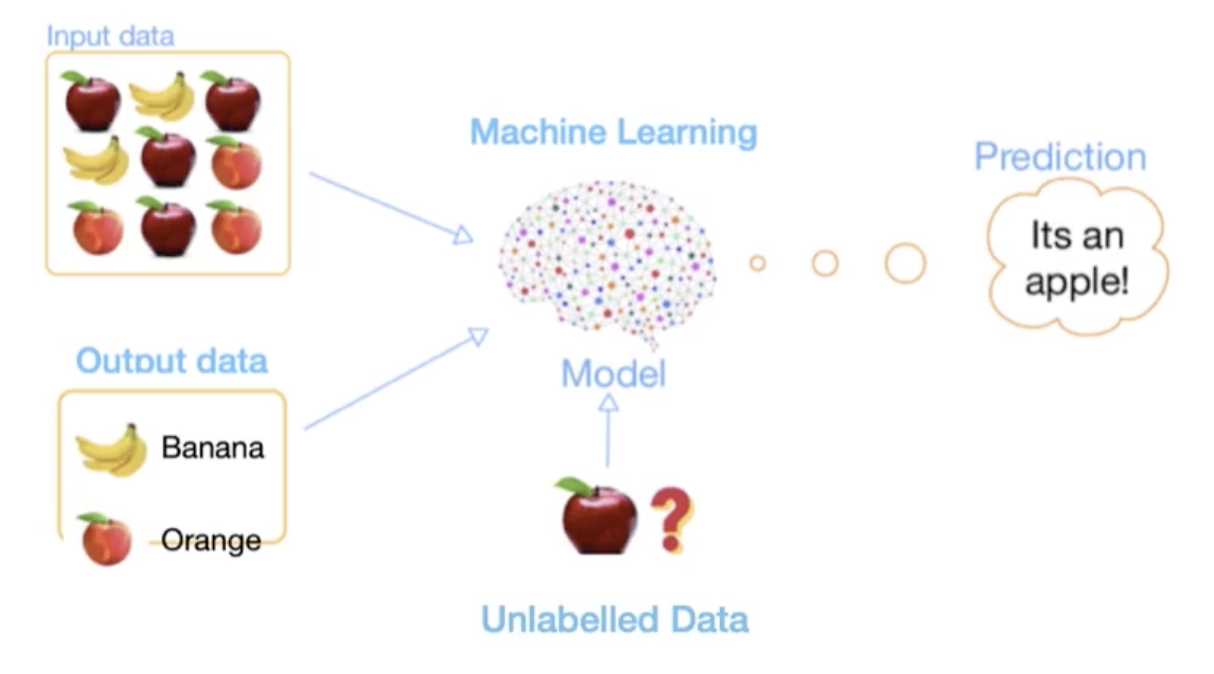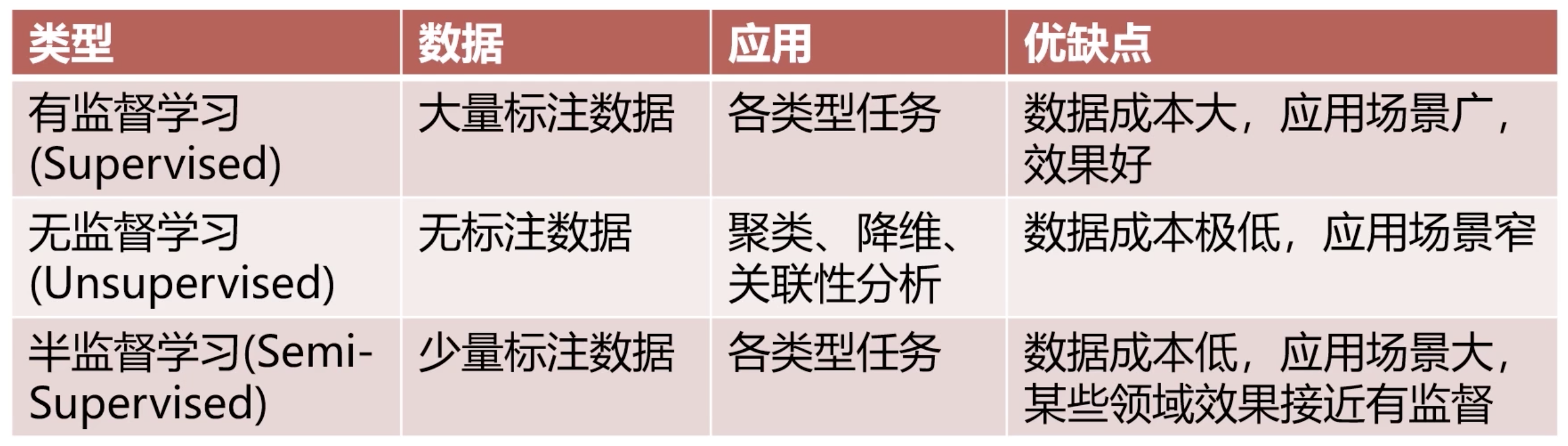1. 视频理解，
2. 自动驾驶
3. 医疗影像分割
4. 心脏信号分析

• 连续性假设(Continuity Assumption):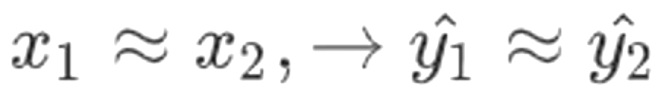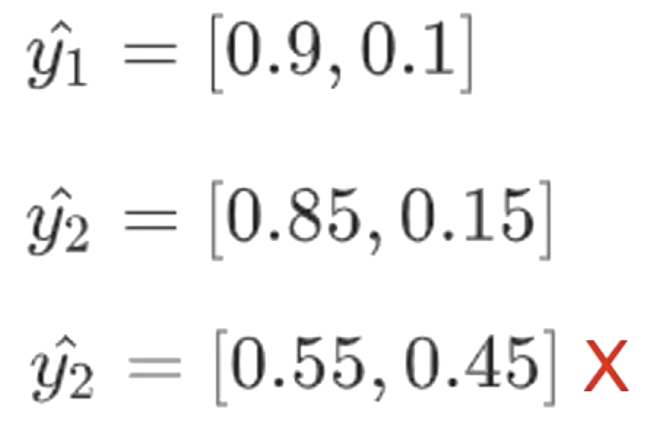• 聚类假设(Cluster Assumption)• 流行假设(Manifold Assumption)
1. 所有数据点都可以被低维流行表达。
2. 相同流行上的数据点，标签一样。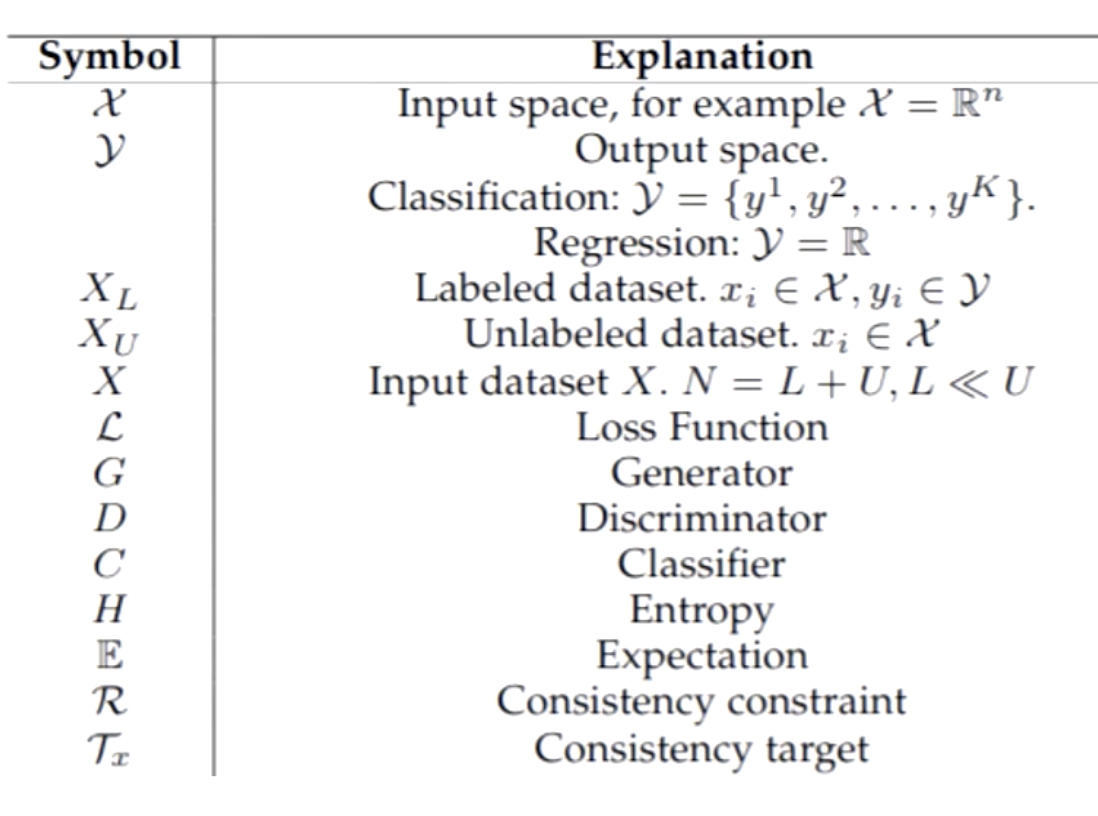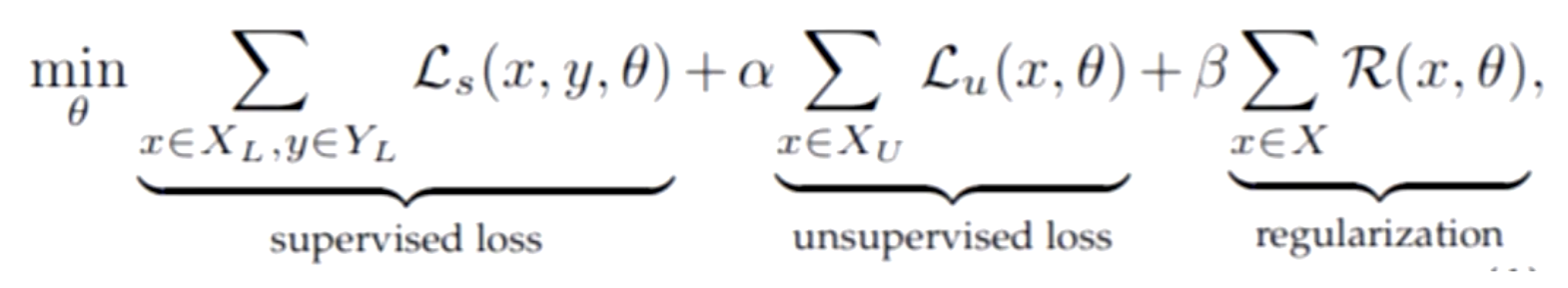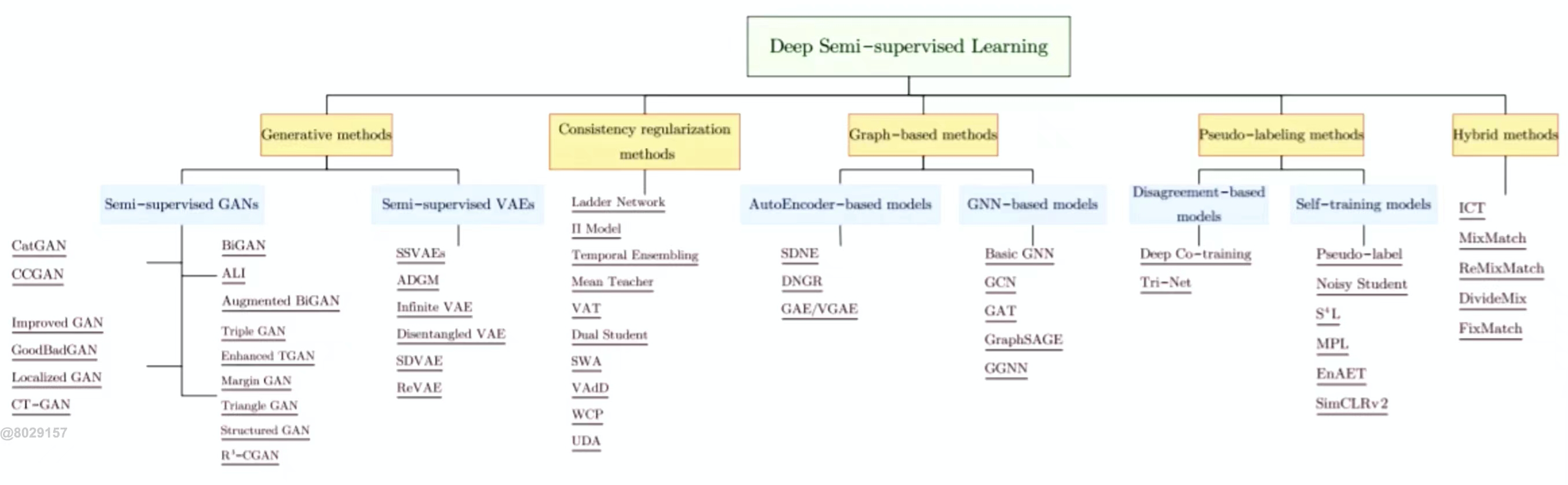• Generative Based:基于生成式网络

1、重用判别器(Re-using Discriminator)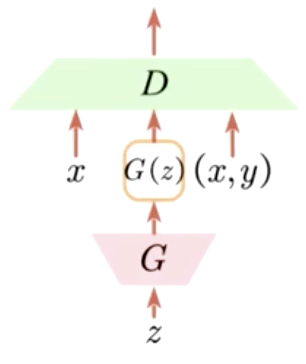2、用于正则化分类器的生成样本(Generated samples to regularize a classifier)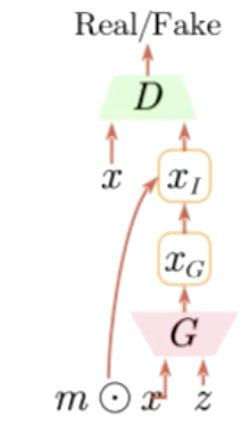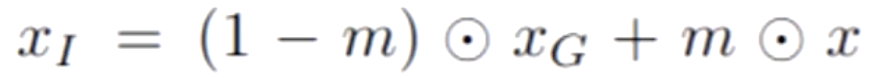3、推理模型(inference model)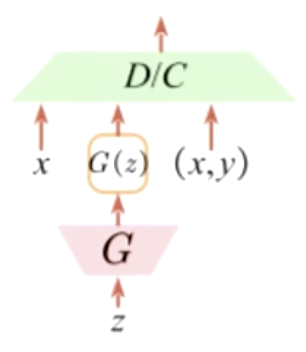4、生成数据(Generate Data)

• Consistency Regularization:一致性正则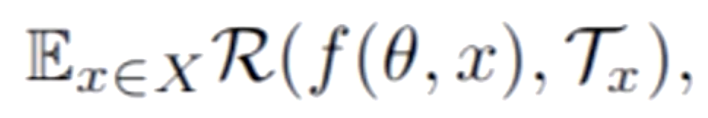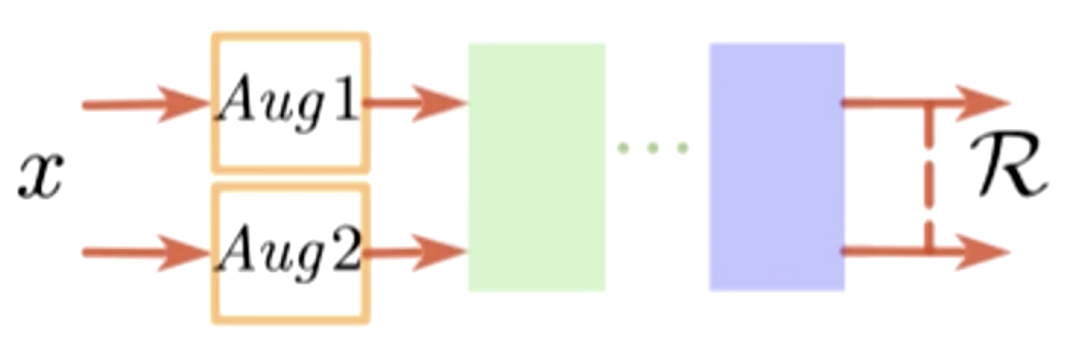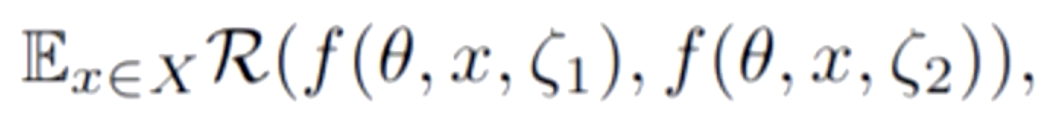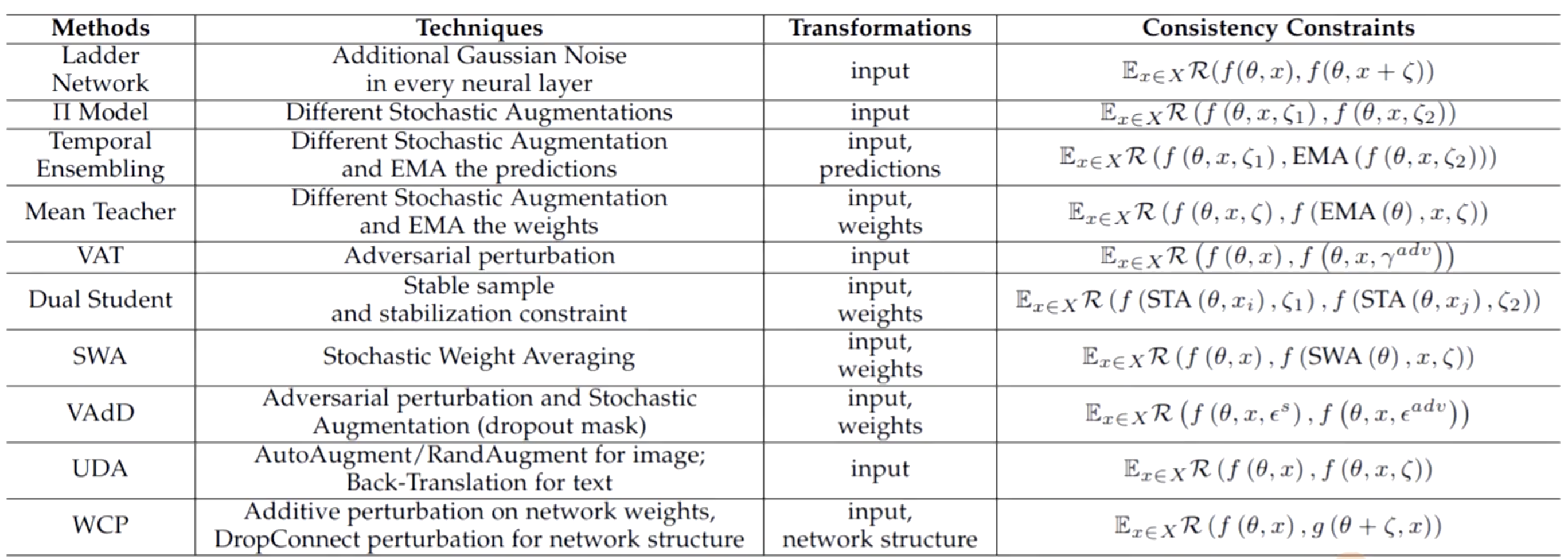• Pseudo-label：伪标签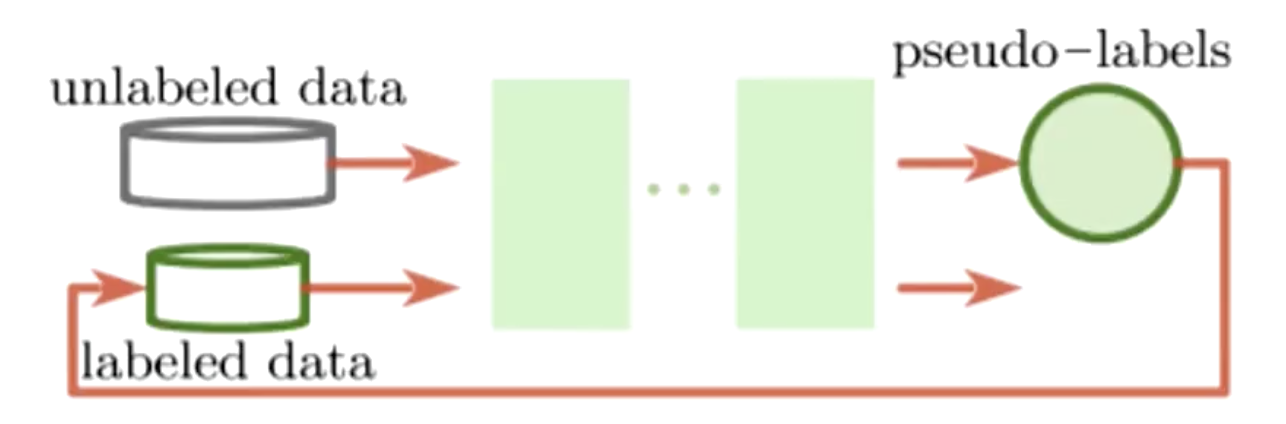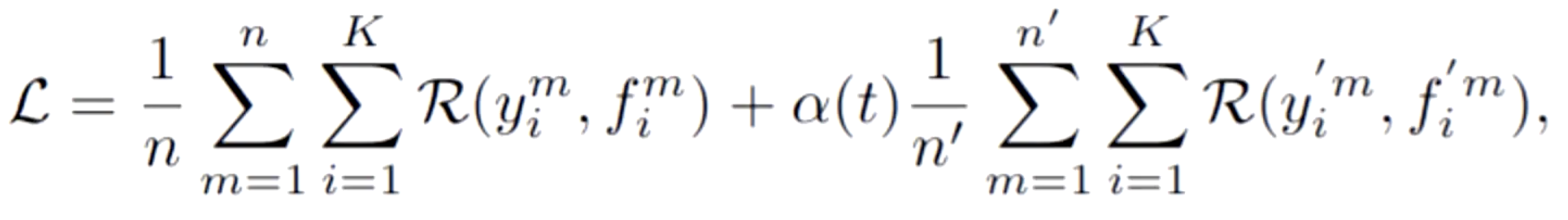1. 伪标签选择不太容易，在模型训练初期，可能是一个不太好的模型，预测出来的标签极有可能是不正确的。如果此时再将预测出来的标签送进模型训练可能会引起进一步的崩溃。
2. 在伪标签损失函数中第二部分有一个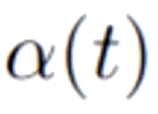，它的意思代表伪标签损失值占整个损失函数多大的比重。而这个的权重值也是很难确定的。如果太小，则未标注数据就失去了作用；太大，如果预测出来的伪标签是不正确的，会导致损失结果难以收敛。

# MixMatch半监督学习

MixMatch结合了之前说的几种方法，用了单个loss，将这几种方式进行合并，如一致性正则，最小化熵，传统正则。它有一个很重要的方法叫MixUp。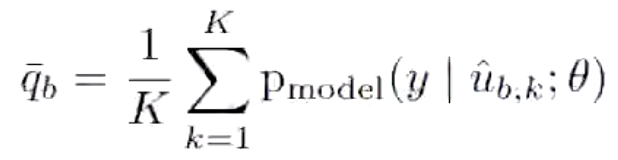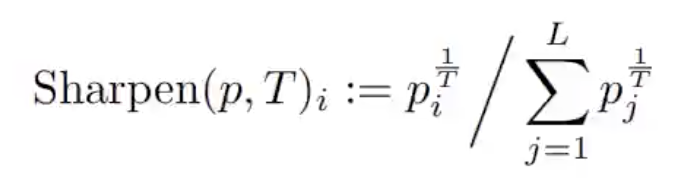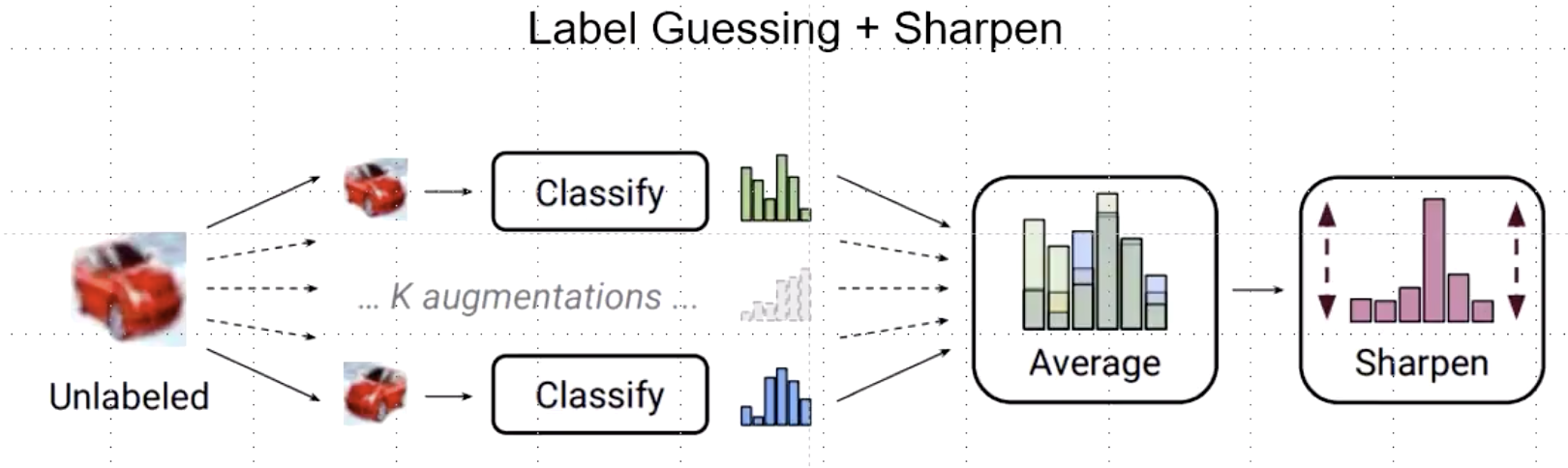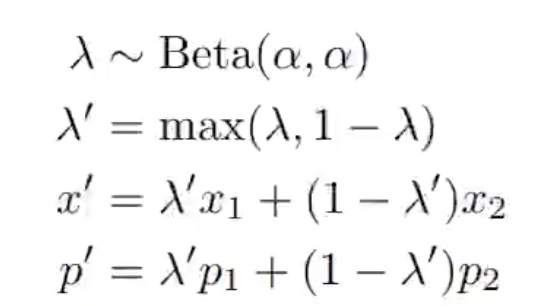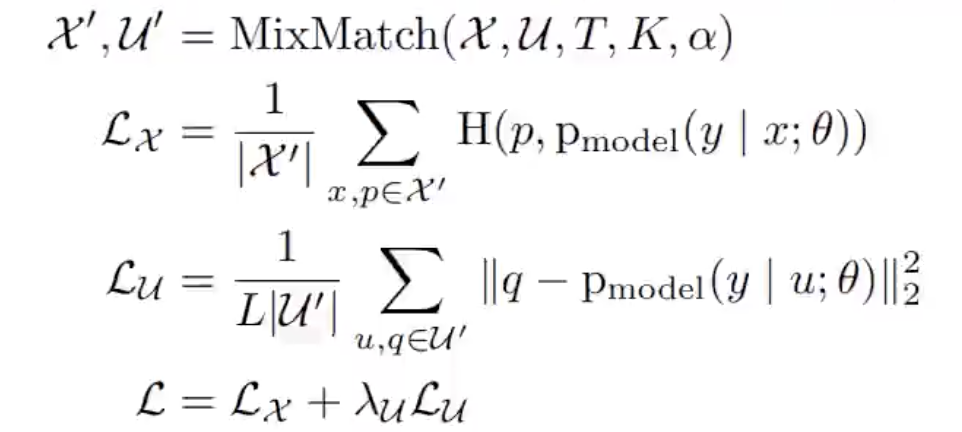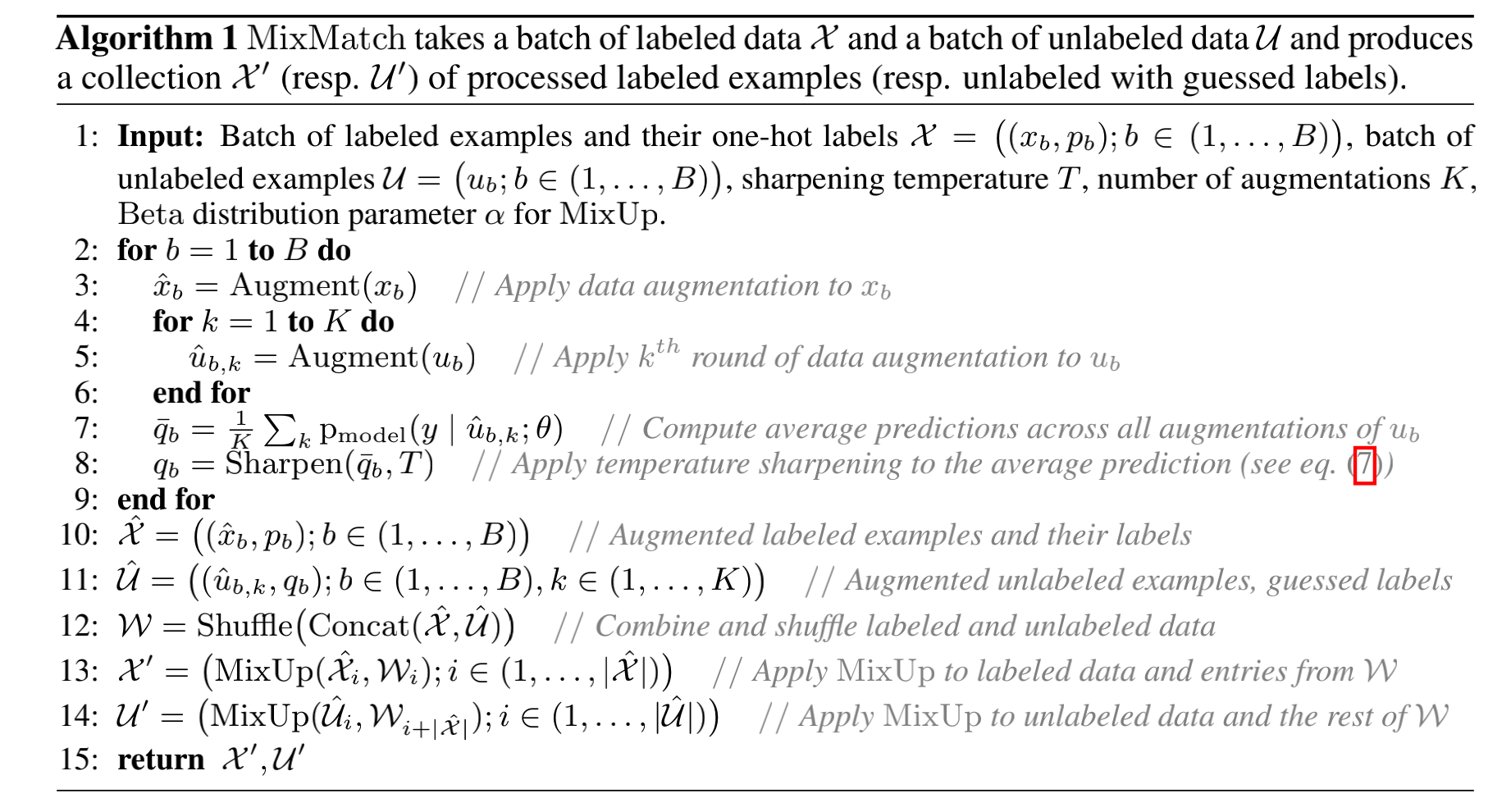import torch

# ################################################################
#                             HyperParameters
# ################################################################
# semi-supervised learning:
#     1. model structure
#     2. hype setting are important!
class Hyperparameters:
# ################################################################
#                             Data
# ################################################################
device = 'cuda' if torch.cuda.is_available() else 'cpu'  # cuda for training, cpu/cuda for inference
classes_num = 10  # 分类数
n_labeled = 250  # 已标记数据总数
seed = 1234

# ################################################################
#                             Model
# ################################################################
T = 0.5  # 锐化温度项(sharpen temperature)
K = 2  # 数据增强次数
alpha = 0.75  # 伪标签损失权值
lambda_u = 75.  # 一致性损失权值
# ################################################################
#                             Exp
# ################################################################
batch_size = 8
init_lr = 0.002
epochs = 1000
verbose_step = 300
save_step = 300

HP = Hyperparameters()

import numpy as np
from torchvision import transforms
import torchvision
import torch

class TransformTwice:
def __init__(self, transform):
self.transform = transform

def __call__(self, inp):
out1 = self.transform(inp)
out2 = self.transform(inp)
return out1, out2

def get_cifar10(root, n_labeled,
transform_train=None, transform_val=None,
# 获取cifar10数据集
# 将该数据集拆分成有标记的训练数据集，无标记的训练数据集和验证集
train_labeled_idxs, train_unlabeled_idxs, val_idxs = train_val_split(base_dataset.targets, int(n_labeled / 10))
train_labeled_dataset = CIFAR10_labeled(root, train_labeled_idxs, train=True, transform=transform_train)
train_unlabeled_dataset = CIFAR10_unlabeled(root, train_unlabeled_idxs, train=True, transform=TransformTwice(transform_train))

print(f"#Labeled: {len(train_labeled_idxs)} #Unlabeled: {len(train_unlabeled_idxs)} #Val: {len(val_idxs)}")
return train_labeled_dataset, train_unlabeled_dataset, val_dataset, test_dataset

def train_val_split(labels, n_labeled_per_class):
labels = np.array(labels)
train_labeled_idxs = []
train_unlabeled_idxs = []
val_idxs = []

for i in range(10):
idxs = np.where(labels == i)
np.random.shuffle(idxs)
train_labeled_idxs.extend(idxs[:n_labeled_per_class])
train_unlabeled_idxs.extend(idxs[n_labeled_per_class:-500])
val_idxs.extend(idxs[-500:])
np.random.shuffle(train_labeled_idxs)
np.random.shuffle(train_unlabeled_idxs)
np.random.shuffle(val_idxs)

return train_labeled_idxs, train_unlabeled_idxs, val_idxs

cifar10_mean = (0.4914, 0.4822, 0.4465)  # equals np.mean(train_set.train_data, axis=(0,1,2))/255
cifar10_std = (0.2471, 0.2435, 0.2616)  # equals np.std(train_set.train_data, axis=(0,1,2))/255

def normalise(x, mean=cifar10_mean, std=cifar10_std):
x, mean, std = [np.array(a, np.float32) for a in (x, mean, std)]
x -= mean*255
x *= 1.0/(255*std)
return x

def transpose(x, source='NHWC', target='NCHW'):
return x.transpose([source.index(d) for d in target])

return np.pad(x, [(0, 0), (border, border), (border, border)], mode='reflect')

"""Crop randomly the image.

Args:
output_size (tuple or int): Desired output size. If int, square crop
"""

def __init__(self, output_size):
assert isinstance(output_size, (int, tuple))
if isinstance(output_size, int):
self.output_size = (output_size, output_size)
else:
assert len(output_size) == 2
self.output_size = output_size

def __call__(self, x):

h, w = x.shape[1:]
new_h, new_w = self.output_size

top = np.random.randint(0, h - new_h)
left = np.random.randint(0, w - new_w)

x = x[:, top: top + new_h, left: left + new_w]

return x

class RandomFlip(object):
"""Flip randomly the image.
"""
def __call__(self, x):
if np.random.rand() < 0.5:
x = x[:, :, ::-1]

return x.copy()

class GaussianNoise(object):
"""Add gaussian noise to the image.
"""
def __call__(self, x):
c, h, w = x.shape
x += np.random.randn(c, h, w) * 0.15
return x

class ToTensor(object):
"""Transform the image to tensor.
"""
def __call__(self, x):
x = torch.from_numpy(x)
return x

class CIFAR10_labeled(torchvision.datasets.CIFAR10):

def __init__(self, root, indexs=None, train=True,
transform=None, target_transform=None,
super(CIFAR10_labeled, self).__init__(root, train=train,
transform=transform, target_transform=target_transform,
if indexs is not None:
self.data = self.data[indexs]
self.targets = np.array(self.targets)[indexs]
self.data = transpose(normalise(self.data))

def __getitem__(self, index):
"""
Args:
index (int): Index

Returns:
tuple: (image, target) where target is index of the target class.
"""
img, target = self.data[index], self.targets[index]

if self.transform is not None:
img = self.transform(img)

if self.target_transform is not None:
target = self.target_transform(target)

return img, target

class CIFAR10_unlabeled(CIFAR10_labeled):

def __init__(self, root, indexs, train=True,
transform=None, target_transform=None,
super(CIFAR10_unlabeled, self).__init__(root, indexs, train=train,
transform=transform, target_transform=target_transform,
self.targets = np.array([-1 for i in range(len(self.targets))])

transform_train = transforms.Compose([
RandomFlip(),
ToTensor(),
])

transform_val = transforms.Compose([
ToTensor(),
])

1. 随着时间流逝，旧的观察值的权重将会呈现指数衰减（Exponential Decay）
2.代表距离当前时刻之前的观察值的权重，那么3. 其中掌控着指数衰减的程度，越大，权重随时间衰减得越快。EMA的迭代定义

1. 等间距的时间序列（等间距：即两个相邻样本之间的时间间隔是不变的）
2. 衰变权重是越大，之前的观察样本获得的权重越小、衰变越多）的迭代公式出发，持续迭代进去，我们得到import torch
import numpy as np

class WeightEMA:
# 指数移动平均，使训练流程更为稳固

def __init__(self, model, ema_model, alpha=0.999):
# 模型
self.model = model
# 影子模型，用于计算指数移动平均参数的模型
self.ema_model = ema_model
# 衰变权重
self.alpha = alpha
# 模型参数
self.params = list(model.state_dict().values())
# 影子模型参数
self.ema_params = list(ema_model.state_dict().values())
# 模型权重值
self.weight_decacy = 0.0004
# 同步模型参数
for param, ema_param in zip(self.params, self.ema_params):
param.data.copy_(ema_param)

def step(self):
# 每一步迭代
for param, ema_param in zip(self.params, self.ema_params):
# 参数类型必须为浮点型
if ema_param.dtype == torch.float32:  # model weights only!
# 获取当前影子模型的参数(带权重)
ema_param.mul_(self.alpha)
# 当前影子模型的参数加上上一步的模型参数(带权重)
# EMA过程结束
# 对整个模型参数进行缩放
param.mul_((1 - self.weight_decacy))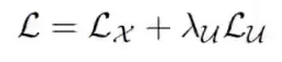def lambda_rampup(step, MAX_STEP=1e6, max_v=75):
"""
求超参数损失函数未标记数据损失函数权重
:param step: 训练步数
:param MAX_STEP: 最大训练步数
:param max_v: 权重最大值
:return: 当前权重值
"""
return np.clip(a=max_v * (step / MAX_STEP), a_min=0., a_max=max_v)

def label_guessing(out_u, out_u2):
"""
经过两次数据增强K=2(default)进行标签猜测
:param out_u: [N, 10], 第一次数据增强的结果
:param out_u2: [N, 10]，第二次数据增强的结果
:return: average label guessing, [N, 10]
[[0.22, 0.32......], => sum = 1.
[0.01, 0.3, 0.03...], => sum = 1.
....]
"""
# 对两次数据增强的结果的后验概率取均值，即除以2
q = (torch.softmax(out_u, dim=-1) + torch.softmax(out_u2, dim=-1)) / 2.
# algorithm 1, line 7
return q

def sharpen(p, T):
"""
锐化，使输出更加尖锐，整体计算方式为对标签猜测后的后验概率求1/T次方再求和
然后再使用标签猜测后的后验概率求1/T次方除以求和的值
:param p: 后验概率
[[0.22, 0.32......], => sum = 1.
[0.01, 0.3, 0.03...], => sum = 1.
....]
:param T: 温度项
:return: sharpened result
"""
p_power = torch.pow(p, 1./T)
return p_power / torch.sum(p_power, dim=-1, keepdim=True)  # [N , 10]

def mixup(x, u, u2, trg_x, out_u, out_u2, alpha=0.75):
"""
mixup: 将打乱后的有标签数据和无标签数据的拼接与有标签数据和无标签数据进行混合
:param x: 有标记数据, [N, 3, H, W]
:param u: 第一种数据增强的无标记数据, [N, 3, H, W]
:param u2: 第二种数据增强的无标记数据, [N, 3, H, W]
:param trg_x: 有标记数据标签,[N, ]=[0, 7, 8...]
:param out_u: 第一种数据增强的猜测标签
:param out_u2: 第二种数据增强的猜测标签
:param alpha: Beta抽样的超参
:return: mixuped result: x: [3*N, 3, H, W], y: [3*N, 10]
"""
batch_size = x.size(0)  # batch size = HP.batch_size
n_classes = out_u.size(1)  # classes number: 10
device = x.device
# [0.1,0.3.0.01.....] dim=10
# [0., 0.,0., 0.,0., 0.,0., 0.,1., 0.,] dim=10
# 将有标记数据的标签转成one-hot形式
trg_x_onehot = torch.zeros(size=(batch_size, n_classes)).float().to(device)
# [0, 0., 0., 0., 0., 0, 0., 0., 0., 0.,]
# trg_x 
# [0, 0., 0., 0., 0., 0, 0., 1., 0., 0.,]
trg_x_onehot.scatter_(1, trg_x.view(-1, 1), 1.)

# 拼接有标记数据和无标记数据
x_cat = torch.cat([x, u, u2], dim=0)
trg_cat = torch.cat([trg_x_onehot, out_u, out_u2], dim=0)
# 获取这两种数据的总量
n_item = x_cat.size(0)  # N*3
# beta抽样
lam = np.random.beta(alpha, alpha)  # eq. (8)
# 获取抽样的值与1-该值的最大值
lam_prime = max(lam, 1 - lam)         # eq. (9)
# 将拼接后的数据随机打乱
rand_idx = torch.randperm(n_item)   # a rand index sequence: [0,2, 1], [1, 0, 2]
x_cat_shuffled = x_cat[rand_idx]    # x2
trg_cat_shuffled = trg_cat[rand_idx]
# 获取输出数据
x_cat_mixup = lam_prime * x_cat + (1 - lam_prime) * x_cat_shuffled    # eq. (9)
# 获取输出标签
trg_cat_mixup = lam_prime * trg_cat + (1 - lam_prime) * trg_cat_shuffled  # eq. (10)

return x_cat_mixup, trg_cat_mixup

def accuracy(output, target, topk=(1, )):
"""
topk 准确率
:param output: 模型输出[N, 10]
:param target: 模型标签[N, ]
:param topk: top1,top3, top5
:return: acc list
"""
maxk = max(topk)  # max k, topk=(1, 3, 5)
batch_size = target.size(0)
# 获取topk的索引
_, pred = output.topk(maxk, 1, True, True)
# 转置
pred = pred.t()  # [maxk, N]
# 获取比较矩阵
correct = pred.eq(target.view(1, -1).expand_as(pred))
res = []
# 获取每一种top的准确率
for k in topk:
correct_k = correct[:k].reshape(-1).float().sum(0)
res.append(correct_k.mul_(100. / batch_size))
return res  # [50, 85, 99]

import torch
import torchvision
from torch import nn
from config import HP

class WideResnet50_2(nn.Module):
def __init__(self):
super(WideResnet50_2, self).__init__()
resnet = torchvision.models.wide_resnet50_2(pretrained=False)
last_fc_dim = resnet.fc.in_features  # defaut imagenet, 1000
fc = nn.Linear(in_features=last_fc_dim, out_features=HP.classes_num)
resnet.fc = fc
self.wideresnet4cifar10 = resnet

def forward(self, input_x):
return self.wideresnet4cifar10(input_x)

import torch
import torch.nn.functional as F
from torch import nn

class MixUpLoss(nn.Module):
def __init__(self):
super(MixUpLoss, self).__init__()

def forward(self, output_x, trg_x, output_u, trg_u):
"""
loss function: 损失函数
:param output_x: 混合后的已标记数据模型输出: [N, 10]
:param trg_x: trg_x: 混合后的已标记数据标签: [N, 10]
:param output_u: 混合后的经过2次数据增强的未标记数据模型输出: [2*N, 10]
:param trg_u:  混合后的经过2次数据增强的未标记数据猜测标签: [2*N, 10]
:return:Lx, Lu
"""
# 对混合后的已标记数据模型输出求交叉熵损失函数
Lx = -torch.mean(torch.sum(F.log_softmax(output_x, dim=-1) * trg_x, dim=-1))  # cross-entropy, supervised loss
# 对混合后的经过2次数据增强的未标记数据模型输出求均方误差损失函数
Lu = F.mse_loss(output_u, trg_u)  # consistency reg
return Lx, Lu

import os
import random
from argparse import ArgumentParser

import torch.cuda
import torch.nn as nn
import torch.optim as optim
import torch.utils.data as data
import torchvision.transforms as transforms
from model import WideResnet50_2
import dataset.cifar10 as dataset
from utils import *
from tensorboardX import SummaryWriter
from config import HP
from loss import MixUpLoss

# 随机种子
torch.manual_seed(HP.seed)
torch.cuda.manual_seed(HP.seed)
random.seed(HP.seed)
np.random.seed(HP.seed)

# 用于训练的数据增强和转化
transform_train = transforms.Compose([
dataset.RandomFlip(),
dataset.ToTensor(),
])

# 用于推理，验证，测试的数据转化
transform_val = transforms.Compose([
dataset.ToTensor(),
])

train_labeled_set, train_unlabeled_set, val_set, test_set = dataset.get_cifar10('./data',
n_labeled=HP.n_labeled,
transform_train=transform_train,
transform_val=transform_val)

logger = SummaryWriter('./log')

# 影子模型
def new_ema_model():
model = WideResnet50_2()
model = model.to(HP.device)
for param in model.parameters():
param.detach_()  # 避免梯度追踪
return model

# 保存模型参数
def save_checkpoint(model_, ema_model_, epoch_, optm, checkpoint_path):
save_dict = {
'epoch': epoch_,
'model_state_dict': model_.state_dict(),
'ema_model_state_dict': ema_model_.state_dict(),
'optimizer_state_dict': optm.state_dict(),
}
torch.save(save_dict, checkpoint_path)

# 评价
model_.eval()
sum_loss = 0.
acc1, acc5 = 0., 0.
inputs_x, trg_x = batch
inputs_x, trg_x = inputs_x.to(HP.device), trg_x.long().to(HP.device)
out_x = model_(inputs_x)  # model inference
top1, top5 = accuracy(out_x, trg_x, topk=(1, 5))
acc1 += top1
acc5 += top5
sum_loss += crit(out_x, trg_x)  #计算loss
model_.train()
return acc1, acc5, loss

def train():
parser = ArgumentParser(description='Model Training')
'--c',
default=None,
type=str,
help='train from scratch or resume from checkpoint'
)
args = parser.parse_args()

# 新建模型和影子模型
model = WideResnet50_2()
model = model.to(HP.device)
ema_model = new_ema_model()
# 将模型和影子模型送入指数移动平均
model_ema_opt = WeightEMA(model, ema_model)

# loss
criterion_val = nn.CrossEntropyLoss()  # for eval
criterion_train = MixUpLoss()   # for training

opt = optim.Adam(model.parameters(), lr=HP.init_lr, weight_decay=0.001)  # optimizer with L2 regular

start_epoch, step = 0, 0
if args.c:  # 读取保存的模型
start_epoch = checkpoint['epoch']
print('Resume From %s.' % args.c)
else:
print('Training from scratch!')

model.train()
eval_loss = 0.

# train loop
for epoch in range(start_epoch, HP.epochs):
print('Start epoch: %d, Step: %d' % (epoch, n_unlabeled))
for i in range(n_unlabeled):  # one unlabeled data turn as an epoch
# inputs_x: [N, 3, H, W], trg_x: [N,]
inputs_x, trg_x = next(iter(labeled_trainloader))  # 获取一个batch的已标记数据和标签
# inputs_u / inputs_u2 -> [N, 3, H, W]
(inputs_u, inputs_u2), _ = next(iter(unlabeled_trainloader))  # 获取一个batch的未标记数据(两份)
inputs_x, trg_x, inputs_u, inputs_u2 = inputs_x.to(HP.device), trg_x.long().to(HP.device), inputs_u.to(HP.device), inputs_u2.to(HP.device)

# 对未标记数据进行标签猜测并锐化
out_u = model(inputs_u)  # Aug K=1, inference [N, 10]
out_u2 = model(inputs_u2)  # Aug K=2, inference [N, 10]
q = label_guessing(out_u, out_u2)  # average post distribution [N, 10]
q = sharpen(q, T=HP.T)  # [N, 10],

# 对已标记数据和标签以及未标记数据和猜测标签进行混合得到混合后的数据和标签
# mixuped_x: [3*N, 3, H, W], mixuped_out: [3*N, 10]
mixuped_x, mixuped_out = mixup(x=inputs_x, u=inputs_u, u2=inputs_u2, trg_x=trg_x, out_u=q, out_u2=q)

# 对混合后的数据进行前向推理
mixuped_logits = model(mixuped_x)  # [3*N, 10]
logits_x = mixuped_logits[:HP.batch_size]  # [N, 10]
logits_u = mixuped_logits[HP.batch_size:]  # [2*N, 10]

# 获取已标记数据和未标记数据的损失函数
loss_x, loss_u = criterion_train(logits_x, mixuped_out[:HP.batch_size], logits_u, mixuped_out[HP.batch_size:])
# 合并成总的损失函数
loss = loss_x + lambda_rampup(step, max_v=HP.lambda_u) * loss_u  # eq. (5)
# 对损失函数进行反向传播
loss.backward()
opt.step()
# 进行指数移动平均的模型参数计算
model_ema_opt.step()

if not step % HP.verbose_step:  # 模型评价
acc1, acc5, eval_loss = evaluate(model, val_loader, criterion_val)

if not step % HP.save_step:  # 保存模型参数
model_path = 'model_%d_%d.pth' % (epoch, step)
save_checkpoint(model, ema_model, epoch, opt, os.path.join('./model_save', model_path))

print('Epcoh: [%d/%d], step: %d, Train Loss: %.5f, Dev Loss: %.5f, Acc1: %.3f, Acc5: %.3f'%
(epoch, HP.epochs, step, loss.item(), eval_loss, acc1, acc5))
step += 1
logger.flush()
logger.close()### 作者的其它热门文章

1
1 收藏

0 评论
1 收藏
1